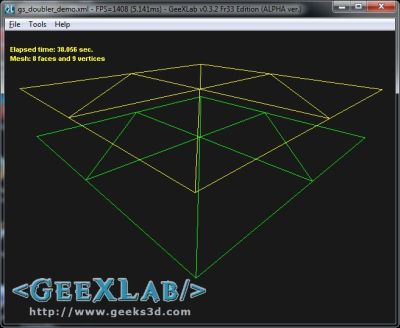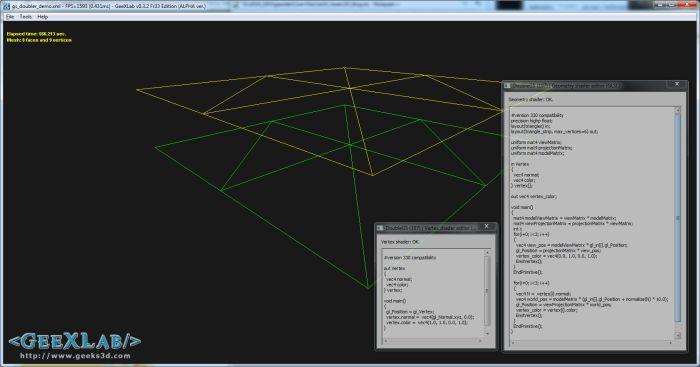# Simple Introduction to Geometry Shaders in GLSL (Part 2)Content:

You missed the first part of this introduction to GS? No problem, you can find it HERE.

## 1 – Doubling the geometry with the geometry shader

Let’s see a more interesting use of the GS: a geometry doubler. This geometry doubler duplicates the input primitives and translates them along the vertex normal.The GS doubler demo in GeeXLab – green: input geometry – yellow: duplicated geometry

Like in the first example, the GLSL program includes a VS, A GS and a PS.

The geometry doubler allows us to see how to pass data from vertex shader to geometry shader.

```#version 330 compatibility
out vec4 normal;
void main()
{
gl_Position = gl_Vertex;
normal =  vec4(gl_Normal.xyz, 0.0);
}
```

As you can see, this vertex shader is rather mininal (a pass-trought VS). Why? because the vertex transformation will be done in the geometry shader.

In this vertex shader, normal is a vertex attribute and is sent to the geometry shader like the built-in gl_Position. Now let’s see how to read the vertex normal in the GS.

```#version 330 compatibility

layout(triangles) in;
layout(triangle_strip, max_vertices=6) out;

uniform mat4 viewMatrix;
uniform mat4 projectionMatrix;
uniform mat4 modelMatrix;

in vec4 normal[];

out vec4 vertex_color;

void main()
{
mat4 modelViewMatrix = viewMatrix * modelMatrix;
mat4 viewProjectionMatrix = projectionMatrix * viewMatrix;
int i;

//====== First triangle - identical to the input one.
//
for(i=0; i<3; i++)
{
vec4 view_pos = modelViewMatrix * gl_in[i].gl_Position;
gl_Position = projectionMatrix * view_pos;
vertex_color = vec4(0.0, 1.0, 0.0, 1.0);
EmitVertex();
}
EndPrimitive();

//====== Second triangle - translated version of the
// input triangle.
//
for(i=0; i<3; i++)
{
vec4 N =  normal[i];
vec4 world_pos = modelMatrix * (gl_in[i].gl_Position + normalize(N) * 10.0);
gl_Position = viewProjectionMatrix * world_pos;
vertex_color = vec4(1.0, 1.0, 0.0, 1.0);
EmitVertex();
}
EndPrimitive();
}
```

Remember, we are in a geometry shader: that’s why we retrieve per-vertex normals in an array. There is one normal per vertex and the size of the array is… 3 elements because the GS takes a triangle as input data. The number of elements of the array is automatically set by the GLSL compiler according to the input layout (here a triangle).

The geometry doubler shader outputs two triangles: one the is input triangle and the second one is a new triangle: the double. This second triangle is translated along the vertex normal. The translation is done in object space (object space? refresh your memory with this article about transformation spaces) that’s why we do all the computations in the geometry shader. If you try to translate along the normal in another space (world, view, projection), you will end up with strange results…

In order to tell OpenGL we output two triangles, we must modify a bit the output layout compared to our first GS in the part 1:

```layout(triangle_strip, max_vertices=6) out;
```

The GS outputs now 6 vertices.

We used an array to retrieve the normals in the GS. But if you need to pass more vertex attributes between the VS and the GS, there is a more handy way to do it: by using an interface block. An interface block allows to pack in a structure all vertex attributes and to pass a single array from the VS to the GS:

```#version 330 compatibility

out VertexAttrib
{
vec3 normal;
vec4 color;
} vertex;

void main()
{
gl_Position = gl_Vertex;
vertex.normal =  vec4(gl_Normal.xyz, 0.0);
vertex.color =  vec4(1.0, 1.0, 0.0, 1.0);
}
```

```#version 330 compatibility

layout(triangles) in;
layout(triangle_strip, max_vertices=6) out;

uniform mat4 viewMatrix;
uniform mat4 projectionMatrix;
uniform mat4 modelMatrix;

in VertexAttrib
{
vec3 normal;
vec4 color;
} vertex[];

out vec4 vertex_color;

void main()
{
mat4 modelViewMatrix = viewMatrix * modelMatrix;
mat4 viewProjectionMatrix = projectionMatrix * viewMatrix;
int i;

//====== First triangle - identical to the input one.
//
for(i=0; i<3; i++)
{
vec4 view_pos = modelViewMatrix * gl_in[i].gl_Position;
gl_Position = projectionMatrix * view_pos;
vertex_color = vec4(0.0, 1.0, 0.0, 1.0);
EmitVertex();
}
EndPrimitive();

//====== Second triangle - translated version of the
// input triangle.
//
for(i=0; i<3; i++)
{
vec4 N =  vertex[i].normal;
vec4 world_pos = modelMatrix * (gl_in[i].gl_Position + normalize(N) * 10.0);
gl_Position = viewProjectionMatrix * world_pos;
vertex_color = vertex[i].color;
EmitVertex();
}
EndPrimitive();
}
```

And the last shader of our GLSL program:

```#version 330 compatibility
in vec4 vertex_color;
void main(void)
{
gl_FragColor = vertex_color;
}
```

## 2 – The Demo

 The GeeXLab demo is available in the GLSL_Geometry_Shader/ folder of GeeXLab code sample pack: [download#40#image] This demo requires GeeXLab 0.3.2+ (GS support has been added in the 0.3.x branch of GeeXLab).The GS doubler demo in GeeXLab with live coding

## 5 thoughts on “Simple Introduction to Geometry Shaders in GLSL (Part 2)”

1.LLB

Definitely useful, thanks!

2.YulFi

I like a lot your “Simple Introduction” series. It’s well explained.

3.sqrt[-1]

I agree – these simple intros are a useful reference.

4.baron

I get a compilation erorr, when I’m trying to compile this sample.

error C7533: global variable gl_Vertex is deprecated after version 120

This source is incompatible with 330 version.

5.frca

I do not recommend using compatibility profile. It can lead to problems such as one baron describes.